# Why space has three dimensions

By: Mike Gashler

The space we live in has three dimensions.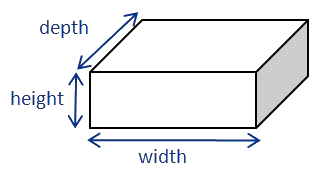But why are there exactly three dimensions? No one knows the answer for sure, so this is an opportunity to speculate!

String theory suggests the universe actually has somewhere from 9 to 11 dimensions, and they exist in a torroid. That means space is like a video game where if you go too far in any direction, you eventually find yourself passing the same places again, like a repeating loop. In the part of the universe where we live, it says three of the dimensions happen to be very large. How large? Like, outer-space large. And all the other dimensions just happen to be very small. How small? Like, so small they are effectively imperceptible.

Well, that's pretty weird, but it didn't really answer the question, did it? So why are there 9 to 11 dimensions, then? And why do we live in a place where three of them are really big and the rest are imperceptibly small?

Next, let's talk about the anthropic principle. Why don't the inhabitants of Mercury or Pluto ever complain about how Earth got all the good properties necessary to support life? Because there aren't any inhabitants on Mercury or Pluto! Why not? Because those places don't have the properties necessary to support life. In other words, if three dimensions were somehow necessary for life, that would explain why we live in a place where there are three dimensions, wouldn't it?

So, let's consider what life might look like in a universe with just two dimensions. All life forms that we know about both consume food and emit waste. But that's a rather difficult thing to pull off in 2-d space. For example, if a creature had both a place where food could enter and exit, that would divide the creature in half!Perhaps a creature could work around this problem by vomiting up its waste instead of pooping. But that's a rather awkward limitation, isn't it? And how many other difficulties will life face in 2-space? It looks to me like life would have a hard time evolving in 2-space. And if 2-space is challenging for life, then 1-space is almost certainly even harder. So, I think it is reasonably clear that if life is going to evolve in some universe, it needs at least two dimensions, and three would be a lot easier. ...but what about four? Would that be even better?

Well, another property life probably needs to thrive is a planet that stays reasonably close to its star. I made a little simulation to test what the orbits of planets would look like in space with varying dimensions.

Below is an orbit in 2-space. (Maybe this could work. At least the planet stays near its star.)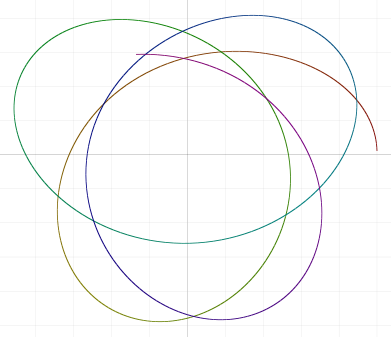Below is an orbit in three-dimensional space. (The reason the path doesn't reconnect to form a perfect ellipse is because my simulation used somewhat coarse time-slices. Reality does a better job of being precise. I could redo this with smaller time-slices, but ...naw, I think it's good enough to illustrate my point.)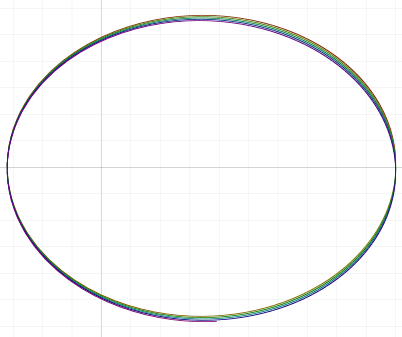Below is what happens in four-dimensional space. (It doesn't even stay in orbit! If we fine-tune the starting conditions more we can make it orbit a bit longer, but even the slightest perturbation will cause it to either drift off into space, or start falling toward the star and then get violently ejected out into space! So solar systems would completely fall apart if there were multiple planets or moons interacting with each other in 4-space.)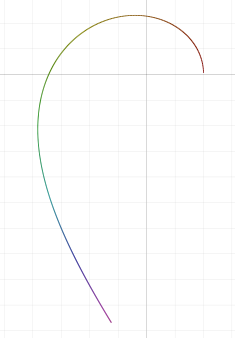And below is what happens in 5-space. (It falls apart even faster!)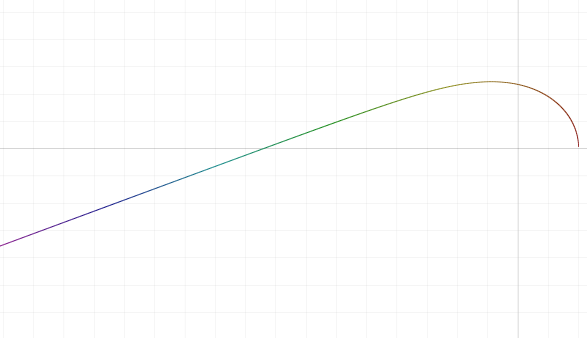So, if we accept string theory, then it makes sense that we would probably live in a place where there are exactly three dimensions of non-degenerate size. (Everywhere else, things just fall apart, so life would never form in the first place.) But should we accept string theory? After all, it makes several non-intuitive assumptions. Torroidal space? At least nine dimensions? What's up with all that weirdness?

Basically, those weird assumptions make certain mathematical equations work out. Incidentally, that's how Maxwell discovered that light was actually electromagnetic radiation--it made some math work. And that's how Einstein put together the theory of Relativity too. So maybe string theory will turn out to be a good description of reality too. And if not, maybe we'll find some way to apply these arguments to whatever theory turns out to be better.

### Technical Notes

• Here's the Python code I wrote to generate those orbital plots. It's not a lot of code, so it shouldn't be too difficult to play with:
```import math

for dims in range(2, 6):
print('\n\nDims=' + str(dims))
grav = 0.001
pos = [1. if i == 0 else 0. for i in range(dims)]
vel = [0.022 if i == 1 else 0. for i in range(dims)]
if dims > 3: vel = 0.033
for i in range(500):
sq_dist = 0.
for j in range(len(pos)):
pos[j] += vel[j]
sq_dist += pos[j] ** 2
dist = math.sqrt(sq_dist)
for j in range(len(pos)):
vel[j] -= pos[j] / dist * grav / (dist ** (dims - 1))
print(str(pos) + ',' + str(pos))```
• This code assumes that the sun is located at the origin and the planet is located one unit away from it. I used an arbitrary gravitational constant and initial velocity. Please feel free to tune them as you wish to produce interesting plots. In the cases of 4 and 5 dimensions, I specially tuned the initial velocity a little better so the planet would survive for longer before drifting away into space. If you remove the line "if dims > 3: vel = 0.033", you'll see that these dimensionalities are even more unstable than my plots seem to imply.
• In geometry, the surface area of a n-sphere is proportional to its radius raised to the (n-1) power. And, presumably for the same reason, in our region of the Universe the force of gravity between two objects is inversely proportional to the square of the distance between them. So my code assumes that in n-space, the force of gravity would be inversely proportional to the distance between the objects raised to the (n-1) power. This is expressed in that code as "grav / (dist ** (dims - 1))" (on the penultimate line). But since I have never actually been to a space with some other dimensionality, I can only assume that this is how physics would really behave there. If you ever happen to travel into some space with a different number of dimensions, I would appreciate if you would please validate this for me.
• Hey, if time is really just another dimension of space, as relativity suggests, then shouldn't the force of gravity in our region of the Universe be inversely proportional to the cube of the distance? Uh oh. It seems like there's something I don't quite understand about this, so be sure to check my work carefully before you accept it.